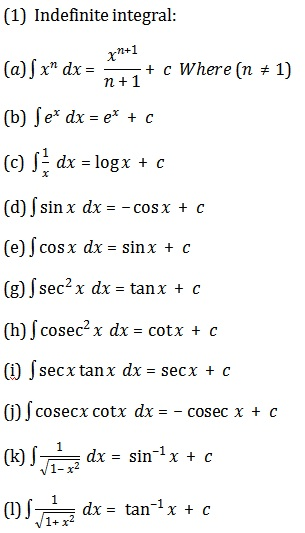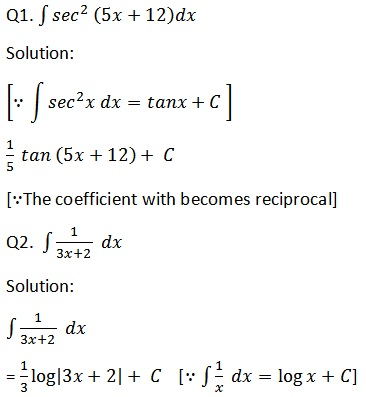Study Materials: CBSE Notes

Our CBSE Notes for Notes 7. Integrals - Introduction | Class 12 Mathematics-II - Toppers Study is the best material for English Medium students cbse board and other state boards students.

Notes ⇒ Class 12th ⇒ Mathematics-II ⇒ 7. Integrals

# Notes 7. Integrals - Introduction | Class 12 Mathematics-II - Toppers Study

Topper Study classes prepares CBSE Notes on practical base problems and comes out with the best result that helps the students and teachers as well as tutors and so many ecademic coaching classes that they need in practical life. Our CBSE Notes for Notes 7. Integrals - Introduction | Class 12 Mathematics-II - Toppers Study is the best material for English Medium students cbse board and other state boards students.

## Notes 7. Integrals - Introduction | Class 12 Mathematics-II - Toppers Study

CBSE board students who preparing for class 12 ncert solutions maths and Mathematics-II solved exercise chapter 7. Integrals available and this helps in upcoming exams 2023-2024.

### You can Find Mathematics-II solution Class 12 Chapter 7. Integrals

• All Chapter review quick revision notes for chapter 7. Integrals Class 12
• NCERT Solutions And Textual questions Answers Class 12 Mathematics-II
• Extra NCERT Book questions Answers Class 12 Mathematics-II
• Importatnt key points with additional Assignment and questions bank solved.

Chapter 7 Mathematics-II class 12

### Introduction class 12 Mathematics-II Chapter 7. Integrals

• Notes 7. Integrals - Introduction | Class 12 Mathematics-II - Toppers Study
• Class 12 Ncert Solutions
• Solution Chapter 7. Integrals Class 12
• Solutions Class 12
• Chapter 7. Integrals Introduction Class 12

## Notes 7. Integrals - Introduction | Class 12 Mathematics-II - Toppers Study

Integrals

Introduction:

(i) Differential Calculus: Differential Calculus is centred on the concept of the derivative. The original motivation for the derivative was the problem of defining tangent lines to the graphs of functions and calculating the slope of such lines.

(ii) Integral Calculus: Integral Calculus is motivated by the problem of defining and calculating the area of the region bounded by the graph of the functions.

(iii) Anti Derivatives: The functions that could possibly have given function as a derivative are called anti derivatives (or primitive) of the function.

(iv) Integration: The formula that gives all these anti derivatives is called the indefinite integral of the function and such process of finding anti derivatives is called integration.

The development of integral calculus arises out of the efforts of solving the problems of the following types:

(a) the problem of finding a function whenever its derivative is given,
(b) the problem of finding the area bounded by the graph of a function under certain conditions.The Fundamental Theorem of Calculus:

There is a connection, known as the Fundamental Theorem of Calculus, between indefinite integral and definite integral which makes the definite integral as a practical tool for science and engineering. The definite integral is also used to solve many interesting problems from various disciplines like economics, finance and probability.

The definite integral is used as a practical tool for:

(i) Science and Ingineering

(ii) Economics, finance and probability.

Integration as an Inverse Process of Differentiation

Integration is the inverse process of differentiation.

Instead of differentiating a function, we are given the derivative of a function and asked to find its primitive, i.e., the original function. Such a process is called integration or anti differentiation.Exercise 7.1Important Study materials for classes 06, 07, 08,09,10, 11 and 12. Like CBSE Notes, Notes for Science, Notes for maths, Notes for Social Science, Notes for Accountancy, Notes for Economics, Notes for political Science, Noes for History, Notes For Bussiness Study, Physical Educations, Sample Papers, Test Papers, Mock Test Papers, Support Materials and Books.Mathematics Class - 11th

NCERT Maths book for CBSE Students.

books

## Study Materials List:

##### Notes ⇒ Class 12th ⇒ Mathematics-II
7. Integrals
8. Application of Integrals
9. Differential Equations
10. Vector Algebra
11. Three Dimensional Geometry
12. Linear Programming
13. Probability

New Books